# Telescope Equations

## Useful Formulas for Exploring the Night Sky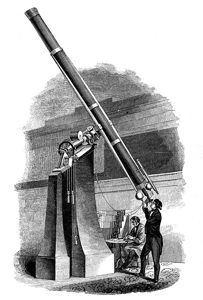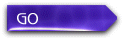IntroductionThe ProcessTerms & SymbolsScope EquationsSpecial Cases

# Introduction

Stars are so unimaginably far away that the light we receive from them arrives in rays that are perfectly parallel. Your eye is designed to focus these parallel rays to a point, allowing you to identify where the light is coming from.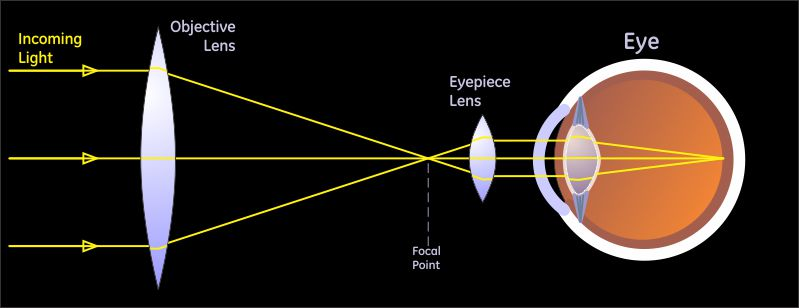A telescope, in its original configuration (refractor), consists of two lenses. The first one, the objective lens, collects light and focuses it to a point. (Note that the objective mirror in a reflecting telescope does exactly the same thing.) The second lens, the eyepiece, catches the light as it diverges away from the focal point and bends it back to parallel rays, so your eye can re-focus it to a point.

Notice how the telescope has taken all the light passing through the objective lens and compressed it down to a column of light that will pass through the pupil of the eye. This is one of the three major tasks of the telescope, the full list being:

• Collect way more light than your eye can, to make a bright image
• Resolve more detail in the image than your eye can without assistance
• Magnify the image so you can see the additional detailThe equations on this page permit you to find just exactly how well the telescope will perform these tasks, and along the way I also show how the tasks are accomplished, by explaining both the theory and the practice. We will be talking specifically about visual observing through the telescope – how the telescope and your eye work together. Understanding photography starts with understanding these ideas, and here we are going to stick to visual observation.

CAUTION - telescope manufacturers will often advertise the magnification of the scope, and give really big, impressive numbers. The problem is that the number is essentially meaningless.

The magnification of a telescope is a combined function of the scope and the eyepiece that is used, so the user can set the magnification to almost any arbitrary value by selecting a suitable eyepiece. Whether the resulting image is clear, or barely visible, depends on other properties of the telescope. Therefore the magnification is not the most important measure of a telescope.

What actually is the most important measure is the diameter of the objective, or more simply the scope diameter, because that determines both the resolving power (the smallest detail you can see) and the light-gathering power (the faintest objects you can see). How the scope diameter determines the performance of your telescope is explained through the equations below.

# The Process

Of course you can use the equations below however you like. Just in case it seems like a lot of equations to you, or maybe looks a bit overwhelming, here's a procedure I can suggest for you. It's in two parts: first finding the intrinsic telescope properties, then determining its operating points.

1.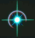Determine the Telescope Properties
2. These are properties of the scope that depend only on the diameter of the objective, so are intrinsic and fundamental to the scope.

1. Resolving Power
• A very simple calculation and very important for determining whether you can split double stars that are close together.

2. Star Magnitude Limit
• Some books will have you calculate the "light grasp", but that tells you nothing by itself. Finding Lmag will tell you which stars you will be able to see in your scope.

3.Determine the Operating Points

The goal is to bracket the range of eyepieces - largest and smallest focal lengths - to use with the scope and determine the performance of the scope at each end of the range.

1. Highest Detail
• Determine maximum magnification: if it is greater than 200, it will be limited by the atmosphere so then call it 200.
• Find the corresponding exit pupil, and from that determine the minimum focal length eyepiece to use, using the two exit pupil equations.
• Find the surface brightness at this operating point from the exit pupil.

2. Highest Brightness
• Determine maximum focal length eyepiece: if it's longer than what is available then set it to the maximum available.
• Find the corresponding exit pupil, and from that determine the minimum magnification, using the two exit pupil equations.
• Find the surface brightness at this operating point from the exit pupil.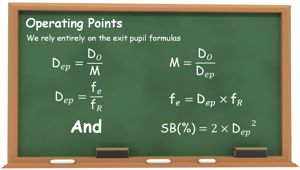## How to Size Up a Scope: Examples

Click on the chalk board to see the process in action with various random interesting examples.

# Terms & Symbols

Symbol Meaning
Dep Diameter of the exit pupil. The exit pupil is where the light leaving the eyepiece converges to its smallest circle -- you find the exit pupil when you bring your eye up to the eyepiece until you can see the whole image.
DO Diameter of the objective. The "objective" can be either the large lens at the front of the telescope (in a refractor) or the large mirror at the back of the telescope (in a reflector).
fe Focal length of the eyepiece. The distance from the center of the eyepiece lens to the point at which light passing through the lens is brought to a focus.
fO Focal length of the objective. The distance from the center of the objective lens (or mirror) to the point at which incoming light is brought to a focus.
fR f-Ratio. Simply the ratio of the focal length to the diameter of the objective, or fO/DO. That means it's the number of lens diameters from the lens to its focal point, as shown below. This is written "f/" and then the value. Often given along with the diameter of the objective to describe the scope.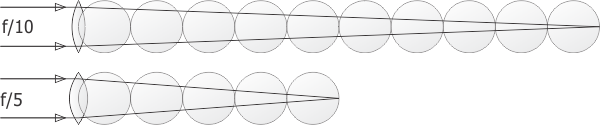FOVe Field of view of the eyepiece. A measure of the area you can see when looking through the eyepiece alone. This is expressed as the angle from one side of the area to the other (with you at the vertex). The two parameters fe and FOVe are the two primary specifications for the eyepiece.
FOVscope Field of view of the scope. Tells you how much of the sky you see in the image in the telescope. This is the distance from one side of the eyepiece image to the other, expressed in degrees or minutes of arc across the sky.
Lmag Star magnitude limit. The faintest star you can see in the scope, expressed in terms of star magnitude. The majority of visible stars have magnitudes in the range of 1-6, where the brightest stars have the lowest magnitude number, near 1, and the faintest you can see by eye are magnitude 6. The scope can show stars as faint as magnitude 16 or even higher (i.e. fainter), depending on its diameter.
M Magnification. The apparent increase in size of an object when looking through the telescope, compared with viewing it directly.
PR Resolving Power. The smallest separation between two stars that can possibly be distinguished with the scope. This is an indication of the finest detail the scope is capable of seeing -- regardless of the magnifying power.
SB Surface Brightness. The brightness, or more correctly the brightness density, of bodies that cover an area, such as planets, nebulae and galaxies. These are distinct from bodies that appear as points, such as stars or asteroids. When an object has area, that area gets larger as magnification is increased, so the light is spread thinner. As a result surface brightness decreases with increasing magnification.

# Scope Equations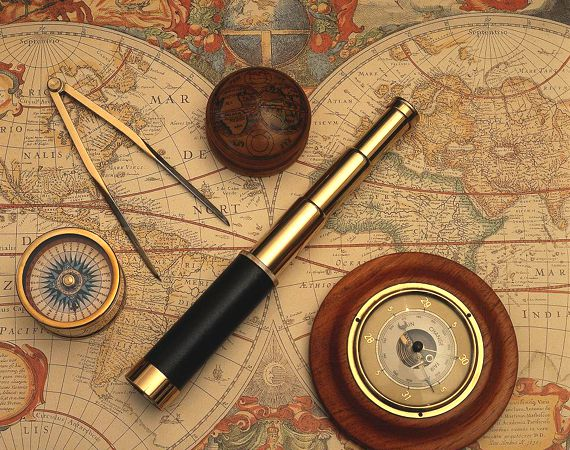So here they are, collected all together. Click on the "Explain This" button next to an equation to see the theory and practice to back it up -- how it came to be, what it means, and how to use it.

Note: I've made these equations as simple and easy to use as possible, so you can do most, if not all, of the calculations in the field in your head.

An important pointer: always work in millimeters. The fact that scope values -- especially the diameter -- are sometimes given in inches and sometimes in centimeters can make things more complicated than they need to be. Millimeters work out really well, so always convert if necessary. Multiply inches by 25 and centimeters by 10 to convert to millimeters.

Term Computed Equation Theory & Practice
Resolving Power (in arcseconds)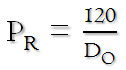Explain This
Magnification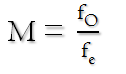orExplain This
Scope Field of ViewExplain This
Image Resolution
What the Eye Resolves at Magnification M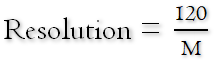Explain This
Diameter of the Exit Pupil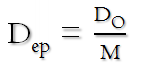or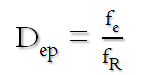Explain This
Focal Length of the Eyepiece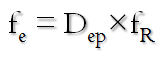Explain This
Star Magnitude Limit
Faintest Star Magnitude the Scope Can See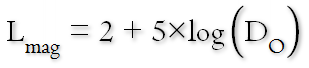Explain This
Surface Brightness
As a Percent of Maximum BrightnessExplain This

# Special Cases

Term Exit Pupil M fe SB Theory & Practice
Minimum Magnification
Highest Brightness the Scope Can Deliver
7 mm DO/7 7×fR 100% Explain This
Wide-Field Magnification
Balance of Performance for Deep Sky Observing
5 mm DO/5 5×fR 50% Explain This
Optimum Magnification
Best Match of Scope & Eye
2 mm DO/2 2×fR 8% Explain This
Maximum Magnification
Highest Detail the Scope Can Deliver
1 mm DO fR 2% Explain This

# Tutorial Presentations

This is how I teach the equations. Note that the full explanation for each slide is in the notes.
Updated 21 April 2019.

This is how I teach the examples. Note that the full explanation for each slide is in the notes.
Updated 11 May 2019.

## Questions

Your questions and comments regarding this page are welcome. You can e-mail Randy Culp for inquiries, suggestions, new ideas or just to chat.
Updated 11 May 2019